### 1.多线和多线段的区别（如图效果，多条平行线构成的多线效果）

### 2.官方对于mline一些常用功能的描述

Start point：起点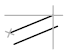Next point：下一个点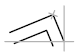Undo: 撤销Next Point操作
Close: 闭合，将所创建的点自动闭合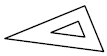Justification

• Top
• Zero
• Bottom
Top: 在光标下方绘制多线，以便具有最大正偏移的线位于指定点处。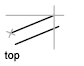Zero: 以光标为中心绘制多线，使 MLSTYLE 元素属性偏移量为 0.0 位于指定点。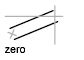Bottom: 在光标上方绘制多线，以便具有最大负偏移的线位于指定点处。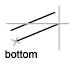Scale：（缩放）

mline.scale = 0; // 表示单根线

mline.scale = 1; // 表示已被的线宽

Controls the overall width of the multiline. This scale does not affect linetype scale.

The scale factor is based on the width established in the multiline style definition. A scale factor of 2 produces a multiline twice as wide as the style definition. A negative scale factor flips the order of the offset line—the smallest on top when the multiline is drawn from left to right. A negative scale value also alters the scale by the absolute value. A scale factor of 0 collapses the multiline into a single line.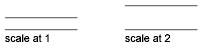Style :指定用于多线的样式。

### 3.相关的代码

``````Mline mine = (Mline)TemporaryEnt;  // 从临时实体中创建
mine.Scale = 0;
if (mine.NumberOfVertices <= 1)
{
mine.AppendSegment(toEyeToWorld(X, Y));
}
else
{
Point3d po = mine.VertexAt(mine.NumberOfVertices - 1);
mine.RemoveLastSegment(out po);
mine.AppendSegment(toEyeToWorld(X, Y));
}
//toEyeToWorld 为通过显示界面返回实际画布的三维坐标值（Z为0）``````

``````            using (var trans = database.TransactionManager.StartTransaction())
{
BlockTableRecord btrec = trans.GetObject(database.CurrentSpaceId, OpenMode.ForWrite) as BlockTableRecord;
// 转换成实体
foreach(ObjectId objid in btrec)
{
Entity ent = trans.GetObject(objid, OpenMode.ForWrite) as Entity;
if (ent.GetType().Name.Contains("Mline"))
{
Mline dbtxta = (Mline)ent;
if (dbtxta.Layer != ori_layer) continue;
MlineStyle mlsta = ((MlineStyle)dbtxta.Style.GetObject(OpenMode.ForRead));  // 多线样式
bool flag = true;
for (int i = 0; i < dbtxta.NumberOfVertices && xyz.Count == dbtxta.NumberOfVertices; i++)
{
// 如果存在一个不满足,则置flag为否
if(xyz[i].X != dbtxta.VertexAt(i).X ||
xyz[i].Y != dbtxta.VertexAt(i).Y ||
xyz[i].Z != dbtxta.VertexAt(i).Z)
{
flag = false;
}
}
// 如果该线段满足位置要求，则复制一个新的放在错误图层中
if (flag)
{
// 通过拷贝的方式在新图层创建这个变量
Mline mline = dbtxta.Clone() as Mline;
btrec.AppendEntity(mline);
mline.Layer = LayerName;    // 添加到图层
}
}

}
// 事务提交
trans.Commit();
// 保存
database.Save();
}``````

xyz结构，只是自己设计的快捷存储了double x,double y,double z这三个变量，没有直接使用Point3D